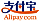Buchcover von Rounding
Buchtitel:

Rounding

Betascript Publishing (05.09.2013 )gutscheinfähig
ISBN-13:

978-613-0-31946-5

ISBN-10:
6130319460
EAN:
9786130319465
Buchsprache:
Englisch
Klappentext:
Please note that the content of this book primarily consists of articles available from Wikipedia or other free sources online. Rounding a numerical value means replacing it by another value that is approximately equal but has a shorter, simpler, or more explicit representation; for example, replacing US\$ 23.4476 by US\$ 23.45, or the fraction 312/937 by 1/3, or sqrt{2} by 1.41. Rounding produces a value that is easier to write and handle than the original, but, on the other hand, it also introduces some round-off error in the result. Rounding is almost unavoidable in many computations — especially when dividing two numbers in integer or fixed point arithmetic; when computing mathematical functions such as square roots, logarithms, and sines; or when using a floating point representation with a fixed number of significant digits. Accurate rounding of mathematical functions is difficult because the number of extra digits that need to be calculated to resolve whether to round up or down is not known in advance. This problem is known as the table-maker's dilemma. Rounding has many similarities to the quantization that occurs when physical quantities must be encoded by numbers or digital signals.
Verlag:
Betascript Publishing
Webseite:
https://www.betascript-publishing.com/
Herausgegeben von:
Lambert M. Surhone, Mariam T. Tennoe, Susan F. Henssonow
Seitenanzahl:
80
Veröffentlicht am:
05.09.2013
Lagerbestand:
Lieferbar
Kategorie:
Mathematik
Preis:
34,00 €
Stichworte:
Round-off Error, Square Root, Logarithm, Quantization Signal Processing, Physical Quantity, Digital Signal, Rational Number, Number System, Vancouver Stock ExchangeKategorien